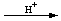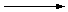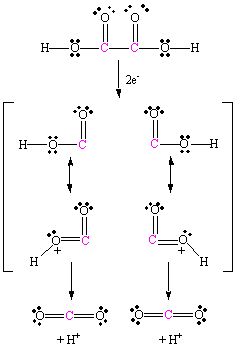Practice Problem 4

We can determine the concentration of an acidic permanganate ion solution by titrating this solution with a known amount of oxalic acid until the charactistic purple color of the MnO4- ion disappears.

H2C2O4(aq) + MnO4-(aq)CO2(g) + Mn2+(aq)

Use the half-reaction method to write a balanced equation for this reaction.

Solution

STEP 1: Write a skeleton equation for the reaction.

H2C2O4 + MnO4-CO2 + Mn2+

STEP 2: Assign oxidation numbers to atoms on both sides of the equation.

 H2C2O4 + MnO4-CO2 + Mn2+ +1 +3 -2 +7 -2 +4 -2 +2

STEP 3: Determine which atoms are oxidized and which are reduced. The only elements that undergo a change in oxidation state are the carbon atoms in oxalic acid and the manganese atom in the MnO4- ion.STEP 4: Divide the reaction into oxidation and reduction half-reactions and balance these half-reactions. This reaction can be divided into the following half-reactions.

 Oxidation: H2C2O4CO2 +3 +4
 Reduction: MnO4-Mn2+ +7 +2

We'll balance the reduction half-reaction first. It takes five electrons to reduce manganese from +7 to +2.

 Reduction: MnO4- + 5 e-Mn2+

Because the reaction is run in acid, we can add H+ ions or H2O molecules to either side of the equation, as needed. There are two hints that tell us which side of the equation gets H+ ions and which side gets H2O molecules. The only way to balance the charge on both sides of this equation is to add eight H+ ions to the left side of the equation.

 Reduction: MnO4- + 8 H+ + 5 e-Mn2+

The only way to balance the number of oxygen atoms is to add four H2O molecules to the right side of the equation.

 Reduction: MnO4- + 8 H+ + 5 e-Mn2+ + 4 H2O

We can now turn to the oxidation half-reaction. We might start by assuming that both of the carbon atoms in oxalic acid end up as carbon dioxide. This is therefore a two-electron oxidation half-reaction.

 Oxidation: H2C2O42 CO2 + 2 e- +3 +4

We can balance both charge and mass by noting that two H+ ions are given off when oxalic acid is oxidized to carbon dioxide,

 Oxidation: H2C2O42 CO2 + 2 e- + 2 H+

as shown in the figure below.STEP 5: Combine the two half-reactions so that electrons are neither created nor destroyed. Five electrons are consumed in the reduction half-reaction and two electrons are given off in the oxidation half-reaction. We can combine these half-reactions so that electrons are conserved by using the lowest common multiple of 5 and 2.

 2(MnO4- + 8 H+ + 5 e-Mn2+ + 4 H2O) + 5(H2C2O42 CO2 + 2 e- + 2 H+) __________________________________________ 2 MnO4- + 16 H+ + 5 H2C2O410 CO2 + 2 Mn2+ + 8 H2O + 10 H+

STEP 6: Balance the remainder of the equation by inspection, if necessary. We can generate the simplest balanced equation for this reaction by subtracting 10 H+ ions from both sides of the equation derived in the previous step.

2 MnO4-(aq) + 5 H2C2O4(aq) + 6 H+(aq)10 CO2(g) + 2 Mn2+(aq) + 8 H2O(l)